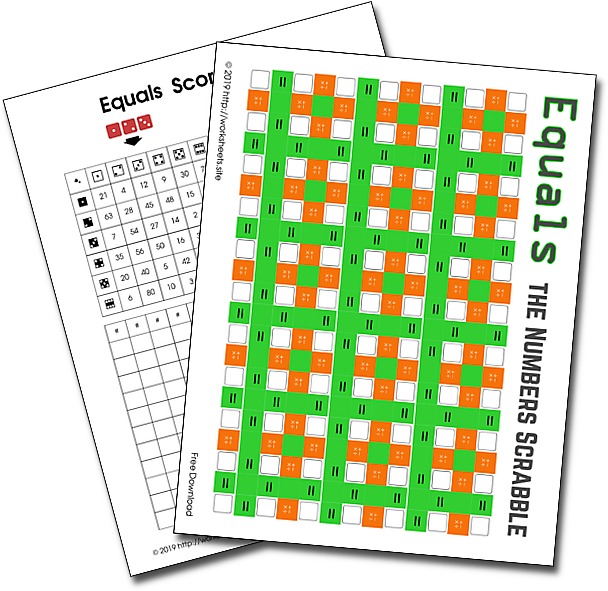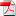# Equals the Numbers Scrabble

An ingenious variation of Scrabble. A popular calculating game for the whole family. Easy to understand game rules because every player knows the basics: the 1x1. Practice the four basic math operations. Math with dice.Equals is a game enjoyed by parents and children alike. It's the world-famous system of crossword games and arithmetic equations combined to score points. All players have a supply of 8 numbers and, with the 4 basic operations, can formulate their equations. After each turn, the number supply is replenished. Different arithmetic operations give you different scores based on their difficulty. Division is awarded a fourfold score, multiplication is triple score, subtraction is double score, and addition is simple score.

## 1. PLAYERS

2-4 players.

Ages 10-99 years.

## 2. GOAL OF THE GAME

To race for the highest score using the number options in your hand and combining them to form valid number equations.

Each player takes 8 numbers and tries to use them in equations. The highest number used in the equation is used as your base score. This number is then multiplied by a factor depending on the operation used. The numbers that were used in the board are replaced by rolling three dice that decided which new number you'll get. The winner is the player scoring the most points when the board is full or when a player reaches the last line of their scoring card.

## 3. HOW TO PLAY

1. Initially, all players get a score sheet and roll three different dice 8 times, to get 8 numbers using the dice tables in the score sheets. They write them down as the first row in their left hand side of their sheets.
2. The player with the highest number begins. The other players follow one by one.
3. The active player tries to place an equation (for example 4 + 4 = 8 or 5 × 7 = 35) in the boxes on the game board sheet.
4. The players can use any operation to construct the equation.
5. When writing on the game board sheet, the following rules apply:
1. The white rounded boxes can only contain numbers.
2. The yellow boxes are for operations.
3. The green boxes with the equal signs connect the calculations with the answer of an equation.
4. The answer should consist of a single number (for example, 3 × 8 = 24, not 3 × 8 = 6 × 4).
5. The calculations can be placed horizontally or vertically. The answer must be to the right or to the bottom.
6. All the numbers must have the same orientation.
7. The first player should write the first numbers (and operation) somewhere in the center of the board.
6. If the player finds an equation, it is scored as follows: The highest number of the equation is multiplied by
1. if it is an addition (sum) (for example: 20 + 30 = 50, that is 50 × 1 = 50 points)
2. if it is a subtraction (subtraction) (for example: 16 - 12 = 4, that is, 16 × 2 = 32 points).
3. for multiplication (for example: 6 × 8 = 48, and then 48 × 3 = 144 points)
4. for divisions (for example, 56 ÷ 8 = 7, then 56 × 4 = 224 points)
7. The players calculates the resulting points and writes them down in the right hand side of the scoring sheet because, in the end they, will be totalled to get the final result.
8. Next, the player crosses off the numbers used in the current score sheet row, copies the remaining numbers to a new row, and replenishes the used numbers with new ones by rolling the three dice, for each missing number, up to 8.
9. Now it's the turn of the next player. You proceed in the same way, but you must place your equation so that at least one number is already on the board. This also applies to the following players (for example, 4 + 4 = 8 + 2 = 10).
10. If players can not think of an equation, they cross off as many numbers as they want, copy the remaining numbers to a new row, and roll dice to get new ones. They miss their turn, the turn passes to the next player.
11. It is valid to make an equation by using a single number, the player gets the points corresponding to the whole equation. In addition, the player can create another equation or renew the numbers as rule J says.

## 4. END GAME

The game ends when the board is full or when players have no more space in their scoring sheets.

The player who has the highest total at the end of the game is the winner.

## 5. REQUIREMENTS

• 3 Dice
• 1 Score card for each player
• 1 Printable Board Game for each game play.

Download the PDF. Use the password worksheets.site to open the PDF file.